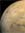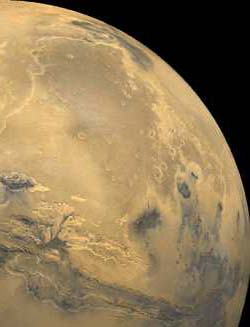# Paint By Numbers

### Activity Summary:

A pencil and paper activity demonstrates how astronomical spacecraft and computers create images of objects in space.

### Objective:

To simulate how light collected from a space object converts into binary data and reconverts into an image of the object.

### Introduction

Discuss with the students how we can't use conventional film on space missions (how would we get it back?). Image data is stored on the satellite and transmitted to Earth as a series of numbers and computers are used to reconstruct the numerical data into the pictures we see.

This activity touches on the following standards:

Mathematics Standards

• Patterns, function, and algebra
• Measurement
• Data analysis, statistics, and probability
• Communications
• Representations
Science Standards
• Evidence, models, and explanation
• Change, constancy, and measurement
• Abilities of technological design

### Procedure

#### Materials (per group of two students)

Transparent grid
Paper grid
Picture of house
Pencil

1. Divide students into pairs.
2. Give one student (A) in each pair a paper copy of the blank grid below. Give the other student (B) in each pair the picture of the house (also below). Instruct student B not to reveal the picture to student A. Also give student B a copy of the grid on transparency film.
3. Explain that the picture is an object being observed at a great distance. It will be scanned by an optical device like those found on some astronomical satellites and an image will be created on the paper. The students with the transparent grid represents the spacecraft and the student with the paper grid represents the radio receiver on the ground and the image-processing computer that will assemble the image of the object.
4. Have Student B place the grid over the picture. Student B should look at the brightness of each square defined by the grid lines and assign it a number according to the chart above the picture. Student B will then call out the number to Student A. If a particular square covers an area of the picture that is both light and dark, student B should estimate its average brightness and assign an intermediate value to the square.
5. After receiving a number from student B, Student A will shade the corresponding square on the grid. If the number is 0, the square should be shaded black. If it is 3, the square should be left as it is.
6. Compare the original picture with the image sketched on the paper.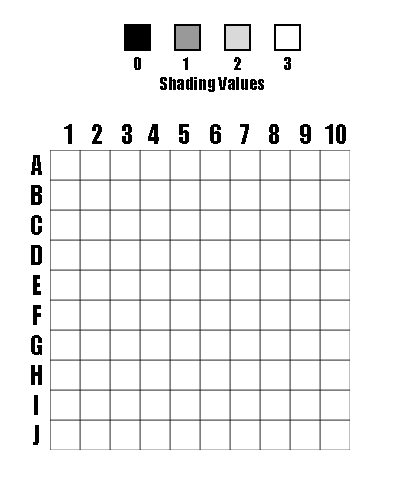100-Pixel Grid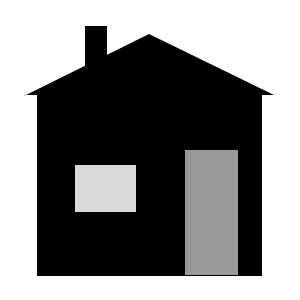### Discussion:

The image created with this activity is a crude representation of the original picture. The reason for this is that the initial grid contains only 100 squares (10x10). If there were more squares, each square would be smaller and the image would show finer detail. You may wish to repeat this activity with a grid consisting of 256 squares (16 x 16) or 400 squares (20 x 20, provided in this lesson plan). Increasing the number of squares will require more class time.

Space-based observatories, like HST, have more than 2.5 million pixels and they are shaded in 256 steps from black to white instead of the 4 shades used in this activity. Color images of an object are created with color filters. The spacecraft observes the object through a red filter, a blue filter, and then a green filter. Each filter creates a separate image, containing different information. These images are then colored and combined in a process similar to color separations used for printing colored magazine pictures.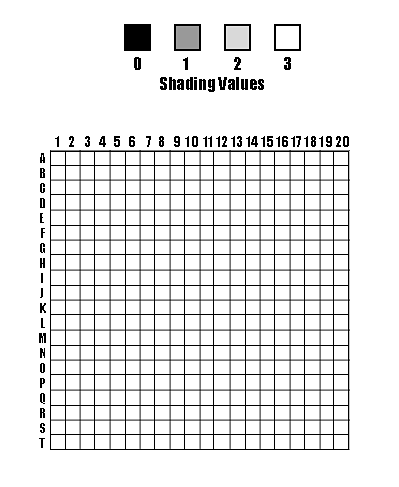Optional 200-Pixel Grid

### Reference:

This activity comes directly from NASA's Space-Based Astronomy Activity Guide for Science, Mathematics, and Technology Education. We thank NASA for the use of this activity.